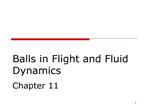DownloadDownload PresentationBalls in Flight and Fluid Dynamics Chapter 11

# Balls in Flight and Fluid Dynamics Chapter 11

Download Presentation## Balls in Flight and Fluid Dynamics Chapter 11

- - - - - - - - - - - - - - - - - - - - - - - - - - - E N D - - - - - - - - - - - - - - - - - - - - - - - - - - -
##### Presentation Transcript

1. 1 Balls in Flight and Fluid Dynamics Chapter 11

2. 2

3. 3 Recall: Fluid = Air is a

4. 4 Viscosity The ability of a fluid to provide resistance against objects moving through it Defined as: ? = coefficient of dynamic viscosity t = shear stress (dv/dy) = rate of shear Given in Pa.s

5. 5 Viscosity cont�d Shear stress: Objects sliding past each other creating friction Shear force divided by area over which it acts

6. 6 Viscosity Causes an irreversible loss of energy Viscosity of water: 0.001 Pa.s

7. 7 Forces on an object in a fluid environment Buoyant forces: Attributable to an object�s immersion in fluid Act vertically upward Dynamic forces: Attributable to an object�s relative motion in fluid Resolved in drag and lift forces

8. 8 Cube in water -- FBD Forces Weight of cube Water pressure on sides Upper force Weight of water above cube Buoyant force Weight of volume of fluid displaced by the object

9. 9 Buoyancy Forces cont�d The size of buoyant force is equal to the weight of volume of fluid displaced by object.

10. 10 Density and Specific Gravity Density is: Given in kg/m3 V = volume m = mass

11. 11 Density and Specific Gravity cont�d Density of water: 1000 kg/m3 Density of air: 1.2 kg/m3 Dependent on temperature and pressure

12. 12 Density and Specific Gravity cont�d Specific gravity: Ratio of weight of an object to weight of an equal volume of water Objects with SG < 1 will float

13. 13 Buoyancy of the Body Lean tissues have densities greater than 1000kg/m3. Fat/air densities less than 1000 kg/m3 Basis for hydrostatic weighing

14. 14 Note: location of Fb depends on varying densities in body. A moment arm can result.

15. 15 Dynamic Fluid Forces Dynamic fluid forces proportional to: Density of fluid Surface area of object Square of velocity of object WRT fluid

16. 16 Dynamic Fluid Forces cont�d Drag force: CD = drag coefficient

17. 17 Relative Velocity Difference between object�s velocity and fluid�s velocity

18. 18 Drag Force Component of dynamic fluid force Acts in opposition to relative motion of object with respect to fluid

19. 19 Drag Force cont�d Two types of drag Surface drag Form drag

20. 20 Surface Drag Molecules slide past the surface and decelerate This also slows down nearby molecules Sum of friction forces

21. 21 Form Drag A fluid molecule bounces off the surface and is then pushed back toward it by other molecules Sum of impact forces

22. 22 Lift Force Acts perpendicular to relative motion of object with respect to fluid CL = coefficient of lift FL = lift force

23. 23 Bernoulli�s Principle Faster-moving fluids exert less pressure laterally than slower-moving fluids Example: airfoil

24. 24 Spin and Magnus Effect Magnus effect: lift forces occur when balls are spinning Backspin Topspin Sidespin

25. 25 Boundary Layer Narrow layer of viscous air lying over object as it travels through the air Reynold�s number describes flow conditions around an object

26. 26 Boundary Layer cont�d Reynold�s number r = radius of ball v = velocity ? = density of air ? = viscosity of air

27. 27 Boundary Layer cont�d Flow conditions can be: Laminar (Re < 500) Turbular (Re > 500)

28. 28

29. 29 Seams and stitches Disrupt boundary layer flow

30. 30 Less drag? Instability in the boundary layer allows faster moving air to mix with the slow moving air near the surface Allows air to flow farther around the ball before the boundary layer separates Smaller turbulent wake, reduced drag